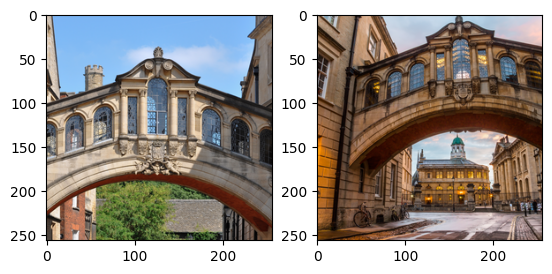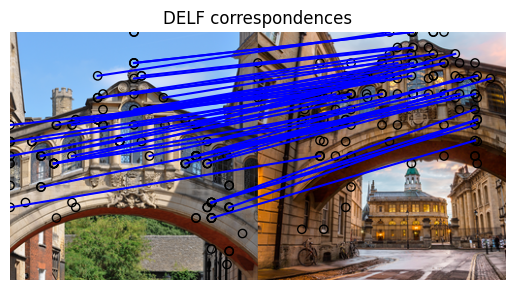# How to match images using DELF and TensorFlow Hub

TensorFlow Hub (TF-Hub) is a platform to share machine learning expertise packaged in reusable resources, notably pre-trained modules.

In this colab, we will use a module that packages the DELF neural network and logic for processing images to identify keypoints and their descriptors. The weights of the neural network were trained on images of landmarks as described in this paper.

## Setup

````pip install scikit-image`
```
``````from absl import logging

import matplotlib.pyplot as plt
import numpy as np
from PIL import Image, ImageOps
from scipy.spatial import cKDTree
from skimage.feature import plot_matches
from skimage.measure import ransac
from skimage.transform import AffineTransform
from six import BytesIO

import tensorflow as tf

import tensorflow_hub as hub
from six.moves.urllib.request import urlopen
``````
```2023-08-19 13:42:36.468771: E tensorflow/compiler/xla/stream_executor/cuda/cuda_dnn.cc:9342] Unable to register cuDNN factory: Attempting to register factory for plugin cuDNN when one has already been registered
2023-08-19 13:42:36.468819: E tensorflow/compiler/xla/stream_executor/cuda/cuda_fft.cc:609] Unable to register cuFFT factory: Attempting to register factory for plugin cuFFT when one has already been registered
2023-08-19 13:42:36.468858: E tensorflow/compiler/xla/stream_executor/cuda/cuda_blas.cc:1518] Unable to register cuBLAS factory: Attempting to register factory for plugin cuBLAS when one has already been registered
WARNING:tensorflow:From /tmpfs/src/tf_docs_env/lib/python3.9/site-packages/tensorflow/python/ops/distributions/distribution.py:259: ReparameterizationType.__init__ (from tensorflow.python.ops.distributions.distribution) is deprecated and will be removed after 2019-01-01.
Instructions for updating:
The TensorFlow Distributions library has moved to TensorFlow Probability (https://github.com/tensorflow/probability). You should update all references to use `tfp.distributions` instead of `tf.distributions`.
WARNING:tensorflow:From /tmpfs/src/tf_docs_env/lib/python3.9/site-packages/tensorflow/python/ops/distributions/bernoulli.py:165: RegisterKL.__init__ (from tensorflow.python.ops.distributions.kullback_leibler) is deprecated and will be removed after 2019-01-01.
Instructions for updating:
The TensorFlow Distributions library has moved to TensorFlow Probability (https://github.com/tensorflow/probability). You should update all references to use `tfp.distributions` instead of `tf.distributions`.
```

## The data

In the next cell, we specify the URLs of two images we would like to process with DELF in order to match and compare them.

### Choose images

``````def download_and_resize(name, url, new_width=256, new_height=256):
path = tf.keras.utils.get_file(url.split('/')[-1], url)
image = Image.open(path)
image = ImageOps.fit(image, (new_width, new_height), Image.LANCZOS)
return image
``````
``````image1 = download_and_resize('image_1.jpg', IMAGE_1_URL)

plt.subplot(1,2,1)
plt.imshow(image1)
plt.subplot(1,2,2)
plt.imshow(image2)
``````
```Downloading data from https://upload.wikimedia.org/wikipedia/commons/2/28/Bridge_of_Sighs%2C_Oxford.jpg
7013850/7013850 [==============================] - 0s 0us/step
14164194/14164194 [==============================] - 1s 0us/step
<matplotlib.image.AxesImage at 0x7f29532a46a0>
```## Apply the DELF module to the data

The DELF module takes an image as input and will describe noteworthy points with vectors. The following cell contains the core of this colab's logic.

``````delf = hub.load('https://tfhub.dev/google/delf/1').signatures['default']
``````
```2023-08-19 13:42:42.256193: E tensorflow/compiler/xla/stream_executor/cuda/cuda_driver.cc:268] failed call to cuInit: CUDA_ERROR_NO_DEVICE: no CUDA-capable device is detected
```
``````def run_delf(image):
np_image = np.array(image)
float_image = tf.image.convert_image_dtype(np_image, tf.float32)

return delf(
image=float_image,
score_threshold=tf.constant(100.0),
image_scales=tf.constant([0.25, 0.3536, 0.5, 0.7071, 1.0, 1.4142, 2.0]),
max_feature_num=tf.constant(1000))
``````
``````result1 = run_delf(image1)
result2 = run_delf(image2)
``````

## Use the locations and description vectors to match the images

### TensorFlow is not needed for this post-processing and visualization

``````match_images(image1, image2, result1, result2)
``````
```Loaded image 1's 233 features